the arc of software bends towards understanding

## Flipping arrows in coBurger King

Category theory crash course for the working Haskell programmer.

A frequent question that comes up when discussing the dual data structures—most frequently comonad—is “What does the co- mean?” The snippy category theory answer is: “Because you flip the arrows around.” This is confusing, because if you look at one variant of the monad and comonad typeclasses:

```class Monad m where
(>>=) :: m a -> (a -> m b) -> m b
return :: a -> m a

(=>>) :: w a -> (w a -> b) -> w b
extract :: w a -> a
```

there are a lot of “arrows”, and only a few of them flipped (specifically, the arrow inside the second argument of the >>= and =>> functions, and the arrow in return/extract). This article will make precise what it means to “flip arrows” and use the “dual category”, even if you don’t know a lick of category theory.

Notation. There will be several diagrams in this article. You can read any node (aka object) as a Haskell type, and any solid arrow (aka morphism) as a Haskell function between those two types. (There will be arrows of different colors to distinguish concepts.) So if I have f :: Int -> Bool, I will draw that as: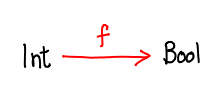Functors. The Functor typeclass is familiar to the working Haskell programmer:

```class Functor t where
fmap :: (a -> b) -> (t a -> t b)
```

While the typeclass seems to imply that there is only one part to an instance of Functor, the implementation of fmap, there is another, almost trivial part: t is now a type function of kind * -> *: it takes a type (a) and outputs a new type (unimaginatively named t a). So we can represent it by this diagram:The arrows are colored differently for a good reason: they are indicating completely different things (and just happen to be on the same diagram). While the red arrow represents a concrete function a -> b (the first argument of fmap), the dashed blue arrow does not claim that a function a -> t a exists: it’s simply indicating how the functor maps from one type to another. It could be a type with no legal values! We could also posit the existence of a function of that type; in that case, we would have a pointed functor:

```class Functor f => Pointed f where
pure :: a -> f a -- aka return
```

But for our purposes, such a function (or is it?) won’t be interesting until we get to monads.

You may have heard of the Functor law, an equality that all Functors should satisfy. Here it is in textual form:

```fmap (g . f) == fmap g . fmap f
```

and here it is in pictorial form: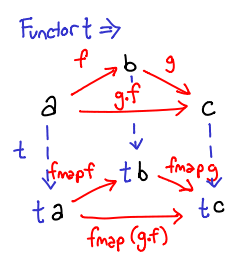One might imagine the diagram as a giant if..then statement: if f, g and g . f exist, then fmap f, fmap g and fmap (g . f) exist (just apply fmap to them!), and they happen to compose in the same way.

Now, it so happens that if we have f :: a -> b and g :: b -> c, g . f is also guaranteed to exist, so we didn’t really need to draw the arrow either. This is such an implicit notion of function composition, so we will take a moment and ask: why is that?

It turns out that when I draw a diagram of red arrows, I’m drawing what mathematicians call a category with objects and arrows. The last few diagrams have been drawn in what is called the category Hask, which has objects as Haskell types and arrows as Haskell functions. The definition of a category builds in arrow composition and identities:

```class Category (~>) where
(.) :: (b ~> c) -> (a ~> b) -> (a ~> c)
id :: a ~> a
```

(you can mentally substitute ~> with -> for Hask) and there are also laws that make arrow composition associative. Most relevantly, the categorical arrows are precisely the arrows you flip when you talk about a dual category.

“Great!” you say, “Does that mean we’re done?” Unfortunately, not quite yet. It is true that the comonad is a monad for an opposite (or dual) category, it is not the category Hask. (This is not the category you are looking for!) Still, we’ve spent all this time getting comfortable drawing diagrams in Hask, and it would be a shame to not put this to good use. Thus, we are going to see an example of the dual category of Hask.

Contravariant functors. You may have heard fmap described as a function that “lifts” functions in to a functorial context: this “functorial context” is actually just another category. (To actually mathematically show this, we'd need to show that the functor laws are sufficient to preserve the category laws.) For normal functors, this category is just Hask (actually a subcategory of it, since only types t _ qualify as objects). For contravariant functors, this category is Hask^op.

Any function f :: a -> b in Hask becomes a function contramap f :: f b -> f a in a contravariant functor:

```class ContraFunctor t where
contramap :: (a -> b) -> t b -> t a
```

Here is the corresponding diagram: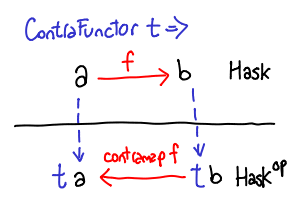Notice that we’ve partitioned the diagram into two sections: one in Hask, and one in Hask^op, and notice how the function arrows (red) flip going from one category to the other, while the functor arrows (blue) have not flipped. t a is still a contravariant functor value.

You might be scratching your head and wondering: is there any instance of contramap that we could actually use? In fact, there is a very simple one that follows directly from our diagram:

```newtype ContraF a b = ContraF (b -> a)
instance ContraFunctor (ContraF a) where
contramap g (ContraF f) = ContraF (f . g)
```

Understanding this instance is not too important for the rest of this article, but interested readers should compare it to the functor on normal functions. Beyond the newtype wrapping and unwrapping, there is only one change.

Natural transformations. I’m going to give away the punchline: in the case of comonads, the arrows you are looking for are natural transformations. What are natural transformations? What kind of category has natural transformations as arrows? In Haskell, natural transformations are roughly polymorphic functions: they’re mappings defined on functors. We’ll notate them in gray, and also introduce some new notation, since we will be handling multiple Functors: subscripts indicate types: fmap_t is fmap :: (a -> b) -> t a -> t b) and η_a is η :: t a -> s a.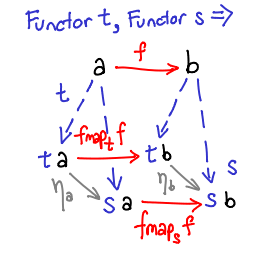Let’s review the three types of arrows flying around. The red arrows are functions, they are morphisms in the category Hask. The blue arrows are indicate a functor mapping between types; they also operate on functions to produce more functions (also in the category Hask: this makes them endofunctors). The gray arrows are also functions, so they can be viewed as morphisms in the category Hask, but sets of gray arrows across all types (objects) in Hask from one functor to another collectively form a natural transformation (two components of a natural transformation are depicted in the diagram). A single blue arrow is not a functor; a single gray arrow is not natural transformations. Rather, appropriately typed collections of them are functors and natural transformations.

Because f seems to be cluttering up the diagram, we could easily omit it: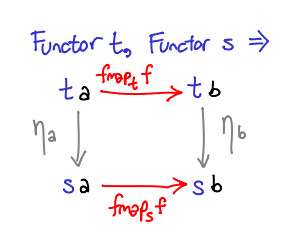```class Monad m where
(>>=) :: m a -> (a -> m b) -> m b
return :: a -> m a
```

You may have heard of an alternate way to define the Monad typeclass:

```class Functor m => Monad m where
join :: m (m a) -> m a
return :: a -> m a
```

where:

```m >>= f = join (fmap f m)
join m = m >>= id
```

join is far more rooted in category theory (indeed, it defines the natural transformation that is the infamous binary operation that makes monads monoids), and you should convince yourself that either join or >>= will get the job done.

Suppose that we know nothing about what monad we’re dealing with, only that it is a monad. What sort of types might we see?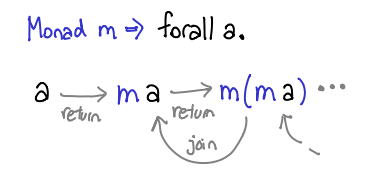Curiously enough, I’ve colored the arrows here as natural transformations, not red, as we have been doing for undistinguished functions in Hask. But where are the functors? m a is trivial: any Monad is also a valid instance of functor. a seems like a plain value, but it can also be treated as Identity a, that is, a inside the identity functor:

```newtype Identity a = Identity a
instance Functor Identity where
fmap f (Identity x) = Identity (f x)
```

and Monad m => m (m a) is just a functor two skins deep:

```fmap2 f m = fmap (fmap f) m
```

or, in point-free style:

```fmap2 = fmap . fmap
```

(Each fmap embeds the function one functor deeper.) We can precisely notate the fact that these functors are composed with something like (cribbed from sigfpe):

```type (f :<*> g) x = f (g x)
```

in which case m :<*> m is a functor.

While those diagrams stem directly from the definition of a monad, there are also important monad laws, which we can also draw diagrams for. I’ll draw just the monad identity laws with f: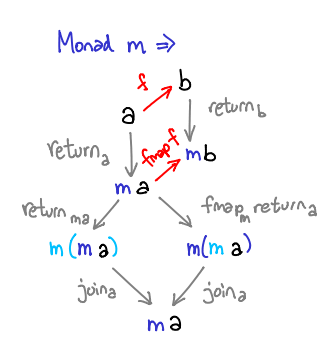return_a indicates return :: a -> m a, and join_a indicates join :: m (m a) -> m a. Here are the rest with f removed: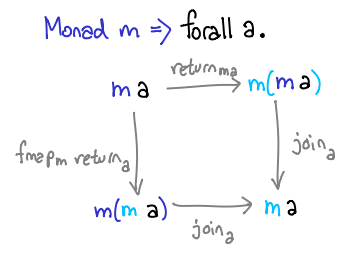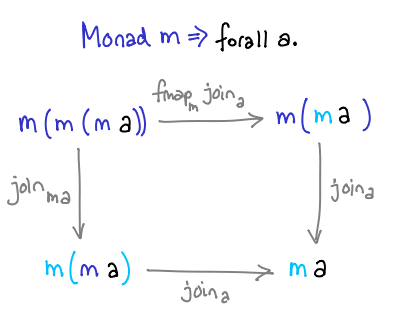You can interpret light blue text as “fresh”—it is the new “layer” created (or compressed) by the natural transformation. The first diagram indicates the identity law (traditionally return x >>= f == f x and f >>= return == f); the second indicates associativity law (traditionally (m >>= f) >>= g == m >>= (\x -> f x >>= g)). The diagrams are equivalent to this code:

```join . return == id == join . fmap return
join . join == join . fmap join
```

Comonads. Monads inhabit the category of endofunctors Hask -> Hask. The category of endofunctors has endofunctors as objects and (no surprise) natural transformations as arrows. So when we make a comonad, we flip the natural transformations. There are two of them: join and return.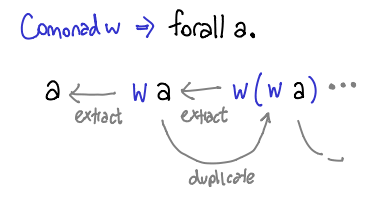Here is the type class:

```class Functor w => Comonad w where
cojoin :: w a -> w (w a)
coreturn :: w a -> a
```

Which have been renamed duplicate and extract respectively.

We can also flip the natural transformation arrows to get our Comonad laws:

```extract . duplicate == id == duplicate . extract
duplicate . duplicate == fmap duplicate . duplicate
```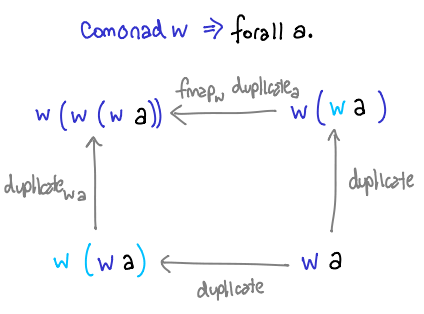Next time. While it is perfectly reasonable to derive <<= from cojoin and coreturn, some readers may feel cheated, for I have never actually discussed the functions from monad that Haskell programmers deal with on a regular basis: I just changed around the definitions until it was obvious what arrows to flip. So some time in the future, I hope to draw some diagrams for Kleisli arrows and show what that is about: in particular, why >=> and <=< are called Kleisli composition.

Apology. It being three in the morning, I’ve managed to omit all of the formal definitions and proofs! I am a very bad mathematician for doing so. Hopefully, after reading this, you will go to the Wikipedia articles on each of these topics and find their descriptions penetrable!

### 23 Responses to “Flipping arrows in coBurger King”

1.Edward Kmett says:

The definition of a Monad in Haskell is a bit muddled by the properties of Hask as a category having arbitrary exponentials.

If you instead consider (=<<) :: (a -> m b) -> (m a -> m b)

Then you can view that as mapping a Kleisli arrow (a -> m b) onto an arrow from (m a -> m b).

Then the dual notion extract :: (w b -> a) -> w b -> w a is reversing the arrows referenced by that mapping. The ‘middle ->’ isn’t logically in the category in question.

But what about (>>=)? Well, by flipping the arguments, now we’ve broken one of those arrows in half, and muddled the existence of the mapping in exchange for obtaining a slightly more pipelined style…. as long as the monad in question is a monad over Hask, rather than some arbitrary Category. Alas, this notion (and parameter order) does not generalize.

I wrote a slightly longer explanation a year or two back for the first meeting of the Boston Haskell user group. Slides are available here: http://comonad.com/haskell/Comonads_1.pdf

2.Edward Kmett says:

[you may need to clean up the formatting on that last post your blog doesn’t like flipped (>>=).]

3.Ben says:

That “instance Comonad w where” should probably be “class”.

4.Edward Z. Yang says:

Ben, thanks, fixed.

Edward, beaten me to the punch as usual. :-) That’s quite a convincing way to think about it, although there is perhaps a slight discomfort at the fact that the connection between your a -> m b to m a -> m b doesn’t smell much like the Kleisli category as described by Wikipedia.

5.Edward Kmett says:

Well, the Kleisli category defined by Wikipedia defines composition in terms of what we call (>=>).

I was just identifying an arrow of the form ‘a -> m b’ as an arrow in the Kleisli category for m, not tying to the notion of the Kleisli category beyond that.

The only real relation to the Kleisli category is that:

(f <=< g) a = f =<< g a

[feel free to reformat, i'm not sure how to get <'s consistently into your blog and can't edit my posts to experiment]

6.solrize says:

7.Edward Z. Yang says:

Oh wow, the Wikibooks article has much more material than I remember it having. They do look like good pairs.

8.Patai Gergely says:

Why do you bring up contravariant functors? They seem out of place in this article.

9.Edward Z. Yang says:

Patai, I originally was hoping there was such a thing as a “cofunctor”, to parallel a “comonad”, but apparently using this name is slightly ambiguous (some people will tell you cofunctors are contravariant functors, some people will tell you those people are confused.) Contravariant functors do have dual categories in them, so they are relevant to the general discussion of duality. :-)

10.Anonymous says:

If flipping the arrow in an object makes a co-object, does flipping them twice get back the original object? That would mean coconuts are the same thing as nuts, cocoa puffs are just puffs, etc. ;-)

11.Edward Z. Yang says:

Yes! More specifically, the dual of a dual category is the original category.

12.Axio says:

When I ask “What is …?” I don’t expect nor particularly logically understand a “Because …” answer!

Also, you wrote :
“The blue arrows are indicate a functor mapping between types… The gray arrows (…) form a natural transformation” immediately followed by
“Blue arrows are not functors; gray arrows are not natural transformations” !!
I believe that last sentence is wrong.
And still don’t get the difference between a natural transformation and an endofunctor…

Cheers.

13.Edward Z. Yang says:

Yes, Axio, you’re right. I should have said a single particular arrow does not indicate a functor. I’ve updated the article accordingly.

The difference between a natural transformation f a -> g a and an endofunctor f a -> f a is two-fold: one is that a natural transformation spans different functors (it transforms from one functor to another) while an endofunctor must map back to itself; the second is that a natural transformation implies the existence of a function f a -> g a; an endofunctor makes no such implication about f a -> f a; the function it defines is slightly different: (f a -> f b) -> f a -> f b (these definitions may seem like mere specializations of id, but if f is known, they don’t necessarily have to be). A little more concisely, natural transformations are functions on values, functors are functions on types. Since most (endo)functors you deal with on a daily basis are also monads, it may seem that the natural transformation a -> f a is fairly certain to exist, but it is by no means a given. What is a function with type “b -> Either a b“? There is no way we can generate a value of type a with just that function.

14. […] but it will take a while before these discussions appear in the F# community. See this article in case you want to know more about […]

15.Boris Nicolaev says:

Probably would be better to add subscript m to the fmap f at the diagram, which illustrates monads identity rules. Thank you very much for this article.

16.Boris Nicolaev says:

Regarding the same diagram, I guess, m in the resulting m a is supposed to be colored light blue. Probably, it is also worth to mention about naturality condition:

fmap f . return = return . f

fmap f . join = join . fmap (fmap f)

17.Edward Z. Yang says:

Sorry, which diagram are you referring to?

18.Boris Nicolaev says:

Here is the modified one: http://i.imgur.com/ULEA5.png

19.Tobin Baker says:

My category theory is weak, but an elementary result in linear algebra is that the dual of a (finite-dimensional) dual vector space is naturally isomorphic to the original vector space. But this is duality of objects, not of categories. Is there any connection with the result on categories that you mention?

20.Edward Z. Yang says:

Tobin: I’m not very familiar with advanced linear algebra, but if the objects are changing, then likely what you have is something like a contravariant functor as opposed to a dualization.

21. […] but it will take a while before these discussions appear in the F# community. See this article in case you want to know more about […]

22. […] but it will take a while before these discussions appear in the F# community. See this article in case you want to know more about […]

23. […] Flipping arrows in coBurger King […]